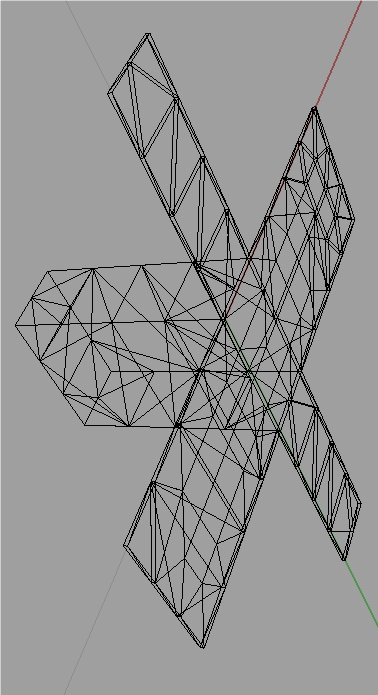Satellite drag

Introduction

The calculation of the drag force acting on a satellite is a classical application of DSMC. Depending on the flight altitude, the flow regime extends from free molecular flow to the slip flow regime. The drag force causes a steady deceleration of the satellite. The drag force depends on the angle of attack, the geometry of the satellite, the density and composition of the surrounding gas and the velocity of the satellite.

When multiple satellites are flying in a formation, the influence among them can be studied.

Example

A triangular surface mesh of the solid body is required for a 3D simulation. The test geometry is a 1 by 2 by 3 units cubesat with deployed solar panels. The mesh below was used as a demonstration. The computational grid is generated automatically. The boundary conditions at the surface and the domain boundaries can be set in the GUI (PIDSMCconfig).The following parameters were used:
100 % N2 atmosphere, rho(inf)=1e-12 kg/m^3, T(inf)=1500 K, V(inf)=7.500 m/s, Tsurface=298 K with diffuse reflection.

The image below shows a cross section through the obtained density field including contour lines at 1, 3 and 5e-12 kg/m^3.As the density of the surrounding gas is very low, there are no molecular collisions. Due to the high velocity, gas is accumulating in front of the solar panels. The satellite is traveling faster than the thermal velocity so that the molecules cannot reach the back of the solar panels.

The result of the simulation also contains the force acting in normal and tangential direction for each surface triangle. From this information the drag force can be calculated for arbitrary angles of attack. In this example the direction of motion is normal to the solar panels. Only the force acting on the front of the solar panel (normal vector 0,0,-1) is considered for simplicity. The obtained drag force is 2.49e-6 N.

The influence of the model for the surface reflection is worth investigating.

The simulation was performed on a workstation with a single XEON E5-2650 and 32GB of RAM. The simulation reached a steady state after 7 hours. The sampling of the surface forces lasted 37 hours. A much shorter sampling time (ca. 8 hours) would probably be sufficient.